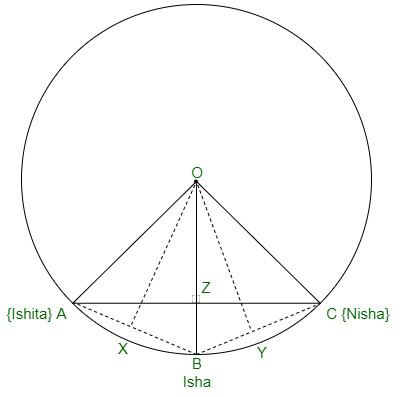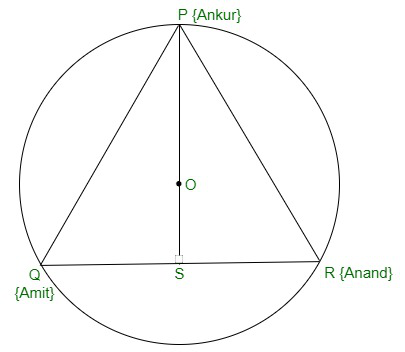Open in App
Not now

# Class 9 RD Sharma Solutions – Chapter 16 Circles – Exercise 16.3

• Last Updated : 19 Oct, 2021

### Question 1. Three girls Ishita, Isha and Nisha are playing a game by standing on a circle of radius 20m drawn in a park. Ishita throws a ball to Isha, Isha to Nisha and Nisha to Ishita. If the distance between Ishita and Isha and between Isha and Nisha is 24m each, what is the distance between Ishita and Nisha?

Solution:Let A, B and C be the position of Ishita, Isha and Nisha respectively.
⇒ XA = AB = 24/2 = 12cm

⇒ OA = OB = OC {As they are radius of the circle.}
In triangle OXA,
⇒ OX2 + XA2 = OA2
⇒ OX2 + 122 = 202
⇒ OX2 = 400 − 144
⇒ OX2 = 256m2
⇒ OX = 16m

Now, let’s take an isosceles triangle ABC as shown above in figure 1.
The isosceles triangle’s altitude divides the base in equal parts.
So in triangle ABC,
⇒ ∠AZB = 90° and AZ = ZC
⇒ Area of triangle OAB = 1/2 × OX × AB { 1/2 × base × height}
⇒ 1/2 × AZ × OB = 1/2 × 16 × 24

⇒ AZ × 20 = 16 × 24
⇒ AZ = 19.2
⇒ AC = 2 × 19.2
⇒ AC = 38.4m
Hence, the distance between Ishita and Nisha is 38.4m.

### Question 2. A circular park of radius 40m is situated in a colony. Three boys Ankur, Amit and Anand are sitting at equal distance on its boundary each having a toy telephone in his hands to talk to each other. Find the length of the string of each phone.

Solution:Given: PQ = QR = RP
Hence, PQR is an equilateral triangle.
Median of an equilateral triangle passes through the circumcenter(O) of the equilateral triangle PQR.
The median of an equilateral triangle intersects each other at the ratio of 2:1.
As PS is the median of equilateral triangle PQR. So,
⇒  OP
OS       1
⇒  40m
OS        1
⇒ OS = 20m
Therefore, PS = OP + OS = 40m + 20m = 60m
In triangle PSR
⇒ PR2 = PS2 + SR2 {By Pythagoras theorem}
⇒ PR2 = 602 + ( QR )2
2
⇒ PR2 = 3600 + ( PR )2 {PR = QR}
4
3 PR2 = 3600
4
⇒ PR2 = 4800
⇒ PR2 = 40√3 m

My Personal Notes arrow_drop_up